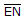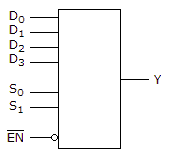# Digital Electronics - Combinational Logic Circuits - Discussion

Discussion Forum : Combinational Logic Circuits - General Questions (Q.No. 4)
4.

For the device shown here, let all D inputs be LOW, both S inputs be HIGH, and theinput be LOW. What is the status of the Y output?LOW
HIGH
Don't Care
Cannot be determined
Explanation:
No answer description is available. Let's discuss.
Discussion:
19 comments Page 1 of 2.

ABHISHEK SHARMA said:   2 years ago
Let EN be a switch, how many and how low or high they are, if EN is switched of then it would definitely be LOW or SWITCHED OFF.

AJAY said:   3 years ago
As EN is low, but in the circuit, the bubble symbol is there, so the device is enabled. As S1, S0 both are high, so it's select D3 as output (which is also low). Therefore, the output of the circuit is low.
(3)

Jyoti said:   4 years ago
Here, EN is low which means the input at EN is low and so the output it is generating to the device is high as it is made using not gate.

SPS said:   5 years ago
It acts as MUX, here s0,s1 are select lines.

EN is high & when,
s0=0,s1=0-->It selects D0
s0=0,s1=1-->It selects D1
s0=1,s1=0-->It selects D2
s0=1,s1=1-->It selects D3
Since s0, s1=1. D3 is selected, which is low. Hence output Y is low.
(3)

MVP said:   7 years ago
Here active low enable used so whatever the input is there that will appear at the output when enable is low.

Praveen said:   7 years ago
How it is low if EN is low?

Dattika said:   7 years ago
Here, whatever value store in D3 that would pass to O/p. So that point how we can say o/p is low?

RAMESH ROY said:   8 years ago
Here inputs that is D low & S be high.

EN=0, then output (Y) is low.

Vatsal Salla said:   9 years ago
Here the circuit works like a mux. S1 S2 as select lines.

So D3 is selected, and will be D3.S1.S2 = LOW.

Nitesh kumar said:   9 years ago
d0 = d1 = d2 = d3 = 0;

s0 = s1 = 1.

For producing output en =0;

When s1 = s2 = 1.

Then d3 is selected.

Hence, y = d3s1s2 = 0;
(1)